CFD modeling of 2D impact with symmetric entry

Roberto Algarín1
Antonio Bula2
Oscar Tascón3

Abstract

The 2D impact phenomenon in calm water, considering symmetric entry with vertical velocity is studied. The analysis was performed by using commercial STAR-CCM+ computational fluid dynamics software. The results obtained from the simulations are pressure distribution and force during impact. The study was carried out for typical planning of boat sections. The results are compared with models and data obtained from some authors and they present very good agreement.

Key words: 2D Impact, CFD modeling, symmetric entry, flow separation.

Resumen

El fenómeno del impacto bidimensional en agua en calma, considerando entrada simétrica con velocidad vertical fue estudiado. El análisis se realizó usando el software comercial CFD STAR-CCM+. Los variables obtenidas de las simulaciones son la distribución de presión y fuerza durante el impacto. El estudio se desarrolló para secciones típicas de botes de planeo. Los resultados son comparados con modelos y valores obtenidos por algunos autores presentando gran similitud.

Date received: May 20th, 2011 - Fecha de recepción: 20 de mayo de 2010
Date Accepted: August 31th, 2011 - Fecha de aceptación: 31 de agosto de 2010

________________________

1 Department of Mechanical Engineering. Universidad del Norte. Barranquilla, Colombia. e-mail: algarinr@uninorte.edu.co, ingmec_83@ yahoo.com.mx
2 Department of Mechanical Engineering. Universidad del Norte, Barranquilla, Colombia. e-mail: abula@uninorte.edu.co
3 Research, Development and Innovation Direction. Science and Technology Corporation for the Development of the Naval, Maritime and Riverine Industry in Colombia. Km 9, Via Mamonal. Cartagena D. T., Colombia. e-mail: otascon@cotecmar.com

............................................................................................................................................................

Symbols

B Beam length.
Cfz Vertical force coefficient.
Cp Pressure coefficient.
fz Vertical Force per unit length.
p Pressure.
t Time.
w Vertical or Impact velocity.

Greek Symbols

τ Time coefficient.
ρ Fluid density.

Introduction

The 2D impact phenomenon has been studied through the application added mass theory, boundary valued problems and CFD applications. Wagner (1932), applied added mass theory to obtain the lift force in a wedge section, as presented in Figure 1. He also evaluated the pressure distribution assuming potential flow and applying energy conservation. Tveitnes (2001), also studied the impact applying added mass theory. He also calculated the lift force, and concluded that the hydrodynamic force experienced by a wedge section under symmetric entry and constant velocity entry, is generated by the variation of mass and flow moment. Moreover, the force exerted during the impact linearly increases as the section submerges and reach the flow separation at the knuckle. This separation occurs when z/d = 2/π . From that point, the moment decreases. The behavior in this zone was obtained empirically, showing that the force remains constant after certain time. The added mass and the flow moment coefficients were determined empirically as a function of the dead rise angle. Caponnetto et al. (2003), generalized the impact for symmetric entry for sections with variable dead rise angle. He also evaluated the flow separation for section including knuckles. Vorus (1996), studied the 2D impact with symmetric entry for a section with variable dead rise angle, solving the problem as a boundary value problem, assuming potential flow. Based on Vorus (1996), Xu et al. (1998) analyzed the 2D impact with asymmetric entry, calculating the pressure, force and roll moment in the section. Seif et al. (2005) simulated the impact using CFD tools for circular and wedge sections, considering symmetric and asymmetric entry over calm water with vertical velocity. The results obtained are very close to experimental results presented in the previous studies. According to his results, the surface tension and viscosity effects can be neglected, but the gravity effect must be considered.

Mathematical Model

In order to present the results, some variables were defined according to the following equations and the geometric characteristics presented in Figure 1: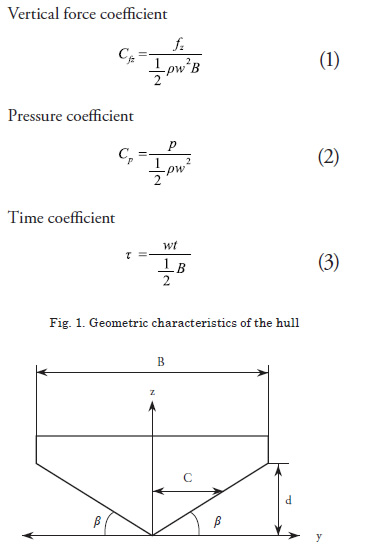Numerical Computation

The conservation of mass and momentum equations were numerically solved. The commercial CFD software STAR-CCM+ was used as a tool to solve the differential equations that govern the phenomenon. The software uses finite volume approximation, and the equations obtained from the discretization procedure are solved using an AMG (Algebraic Multi Grid) solver. The models used to simulate the phenomenon are: multiphase mixture (water-air), inviscid flow, segregated flow and unsteady (implicit method). For the fluids, water was modeled as an incompressible liquid, while air was considered as an ideal gas. In order to confirm that the viscosity and the surface tension could be neglected, some simulations were carried. The conclusions attained are similar to Seif et al. (2005), where the effects of these two variables were discarded. The ratio w /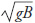took values greater than 0.3.

The size of the computational domain was developed, changing the width and the height in order to model the impact in calm water over an infinite canal. The peak force during the impact was monitored, and the grid size was selected when the variation of the peak force was less than 1%. The final width and height of the domain were 9B to 10B, and 5B to 12.5B, respectively. The time step was also varied considering the impact velocity and it ranged between 0.008 to 0.016 d / w.

The mesh was developed using polyhedral elements, and it was divided in three regions as shown in Figure 2a. The region 1 is mainly water.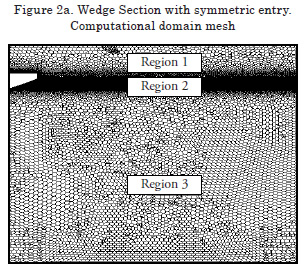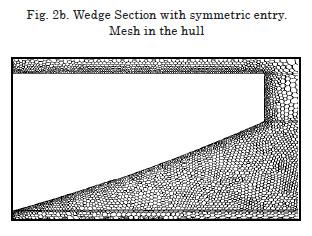Region 2 is a phase mixture, where the hull gets in contact with the water and the air, and the free surface is generated. This area is very important for the study and because of this the mesh was refined as shown in Figure 4b. Region 3 is mainly air.

Figures 2a and 2b show the mesh after a refinement process for a convex section. The base size of the polyhedral element took values ranging between 0.15B to 0.25B. For Region 1 and 3, the values ranged between 0.045B to 0.100B. For Region 2 the values ranged from 0.0075B to 0.020B. The residuals values for the impact force and pressure keel were used as the stop criteria, and the magnitude of the global residual was limited to10- 8, while the maximum numbers of inner iterations in each time step was limited to 30.

Results and Discussion

The boundary conditions for the symmetric entry are: pressure outlet at the top, velocity entry at the bottom, symmetry axis of the section, and wall over the hull.

Figure 3 presents the vertical force coefficient variation for a wedge section with different dead rise angles. The results are compared with Tveitnes (2001) and Caponnetto et al. (2003). The values attained are similar to Tveitnes (2001), presenting a 5% maximum error in the peak force. From Figure 3, it is noticed that z / d = 0 represents the instant when the impact begins. From that point, the vertical force presents a linear behavior increasing up to the point where flow separates from the knuckle, approximately at z / d = 2 / π. After that, the pressure and force decrease to a minimum value.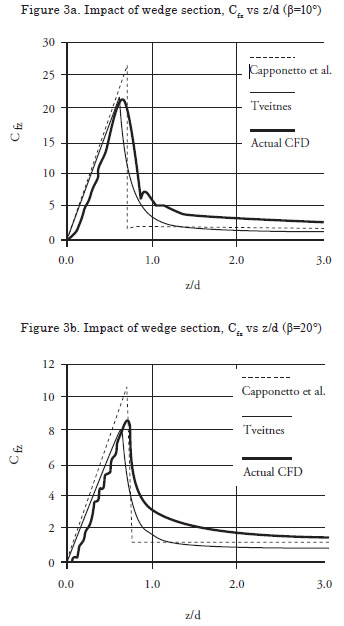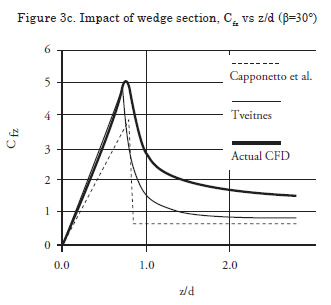Figure 4 presents the pressure coefficient distribution for a wedge section with different dead rise angles. The results attained compare very well to the results presented by Wagner (1932) and Caponnetto et al. (2003). The maximum error on the keel pressure is 12% respect to Caponnetto et al. (2003). The trend shows that the pressure coefficient has a higher value close to the final contact point over the hull. Usually, the hydrodynamic pressure is smaller in the keel , at y / c = 0, and increases as it moves away from this point. At the final contact point y / c = 1, the pressure decreases once again. For a circular section with radius R=5.5m and entry velocity of 10 m/s, the vertical force coefficient was calculated, and the results are presented in Figure 5. It is shown that hydrodynamic force is maximum when the impact begins, z/R=0, and decreases as the section is submerged. The results present a maximum error measured at the peak force, close to 4% when compared to Campbell and Weinberg (1980).

Figure 6 presents concave and convex sections studied by Vorus (1996). Figs. 7 and 8 show the vertical force coefficient and keel pressure coefficient variation with the time for the concave section. The force and pressure coefficients increase when the section submerges up to the point where the flow separates at the knuckle. After this point, both coefficients decrease.

Figures 9 and 10 show the force coefficient at the hull and keel pressure coefficient variation with the time for the convex section. Both coefficients present a trend similar to the concave section. The force increase as the section submerges up to the point where the flow separates at the knuckle, while the peak pressure occurs when impact begins and decrease as the section submerges.

Figures 7 to 10 present a good agreement for the pressure and force distribution before flow separation. Respect to Caponnetto et al. (2003), the maximum error in the peak force is 9% and of the pressure peak is 8%. Respect to Vorus (1996), the main difference is after the flow separates from the knuckle where the simulations show higher value for pressure and force, and the error presents values similar to Caponnetto et al. (2003).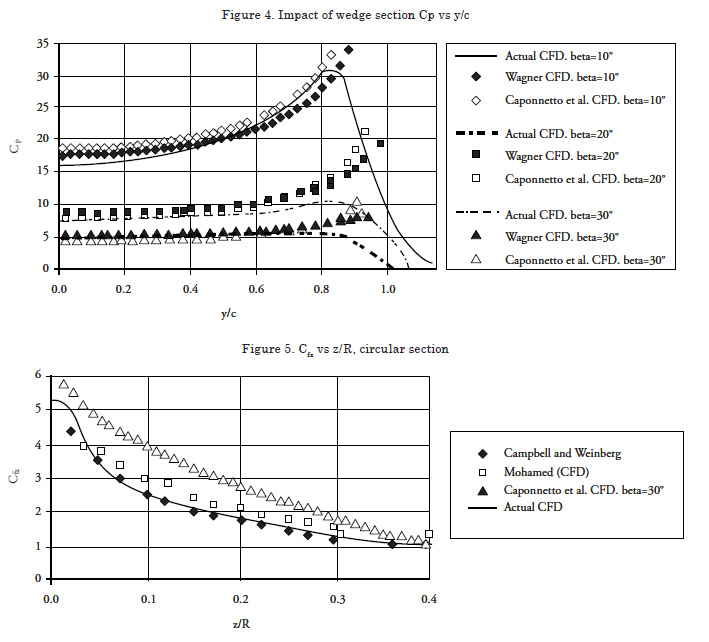Conclusions

The 2D impact was modeled with symmetric entry of wedge sections with variable dead rise angle with CFD software STAR CCM+. The results obtained for the force and pressure coefficient present a good agreement compared to the values reported by Wagner (1932), Caponnetto et al. (2003), Tveitnes (2001), Vorus (1996) and Campbell and Weinberg (1980).aa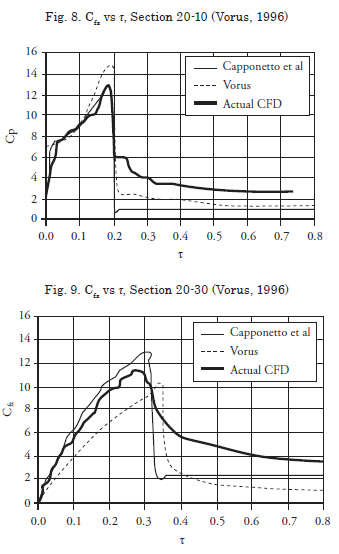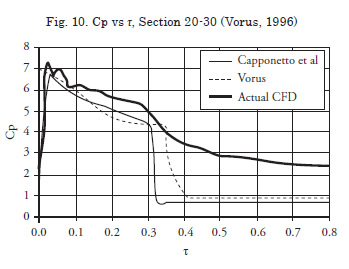References

CAMPBELL, I.M.C. and WEINBERG, P. A., "Measurement of parameters affecting slamming," Wolfson Unit, University of Southampton, Report No. 440, 1980.

CAPONNETTO, M., SÖDING, H., AZCUETA, R. (2003): “Motion Simulations for Planing Boats in Waves”, Ship Technology Research, Vol 50, pp. 182-196.

SEIF, M.S., MOUSAVIRAAD, S.M., SADDATHOSSEINI, S.H. & BERTRAM, V., Numerical Modeling of 2-D Water Impact in One degree of Freedom. En: Síntesis Tecnológica. Noviembre, 2005, vol 2, no 2, p. 79-83.

TVEITNES, T. Application of Added Mass theory in planing [Ph.D. Thesis]. Glasgow: University of Glasgow. Departamento de Arquitectura Naval y Ingeniería Marina, 2001, 339p.

VORUS, W. S. A flat cylinder theory for vessel impact and steady planing resistance. En:Journal of Ship Research. Junio, 1996, vol 40, no 2, p. 89-106.

WAGNER, H. Über stoss – und Gleitvorgänge an der Oberfläshe von Flüssigkeiten. En: Zeitschrift für Angewandte Mathematik und Mechanik. Agosto, 1932, vol 12, no 4, p. 193-215.

XU, L., TROESCH, A.W., and VORUS, W.S. Asymmetric Vessel Impact and Planing Hydrodynamics. En:Journal of Ship Research, Septiembre,1998, vol 42, no 3, p. 187-198.